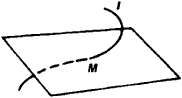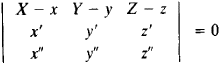# Osculating Plane

(redirected from Contact (mathematics))
Also found in: Dictionary, Thesaurus, Medical, Wikipedia.
Related to Contact (mathematics): Mathematical symbols

## osculating plane

[′äs·kyə‚lād·iŋ ′plān]
(mathematics)
For a curve C at some point p, this is the limiting plane obtained from taking planes through the tangent to C at p and containing some variable point p ′ and then letting p ′ approach p along C.
McGraw-Hill Dictionary of Scientific & Technical Terms, 6E, Copyright © 2003 by The McGraw-Hill Companies, Inc.
The following article is from The Great Soviet Encyclopedia (1979). It might be outdated or ideologically biased.

## Osculating Plane

The osculating plane of a curve l at a point M is the plane having contact of order n ≥ 2 with l at M. The oscillating plane can also be defined as the plane in the limitingFigure 1

position of the plane through three points of l as the points approach M.

From the standpoint of mechanics, the osculating plane can be characterized as the acceleration plane. As a mass point moves arbitrarily along l, the acceleration vector lies in the osculating plane.

Except for special cases, l usually penetrates the osculating plane at M (see Figure 1). If l is defined by the equations x = x(u), y = y(u), and z = z(u), the equation of the osculating plane is of the formHere, X, Y, and Z are the moving coordinates, and x, y, z, x’, y’, z’, x”, y”, and z” are computed at M. If all three coefficients of X, Y, and Z in the equation vanish, the osculating plane is undefined—it can coincide with any plane through the tangent. (See alsoDIFFERENTIAL GEOMETRY.)

### REFERENCE

Rashevskii, P. K. Kurs differentsial’noi geomelrii, 4th ed. Moscow, 1956.